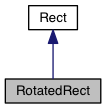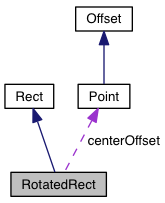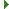PDG engine v0.9.5
RotatedRect Class Reference

rectangle with rotation for 2D coordinate system. More...

Inheritance diagram for RotatedRect:[legend]
Collaboration diagram for RotatedRect:[legend]

## Public Member Functions

RotatedRect (Rect rect=Rect(0, 0), number rotationRadians=0.0, Offset cpOffset=null)

setCenterOffset (Offset cpOffset)

setRotation (number rotationRadians, Offset cpOffset=null)Public Member Functions inherited from Rect
Rect ()

Rect (number w, number h)

Rect (Point topLeft, number w, number h)

Rect (Point leftTop, Point rightBottom)

Rect (number left, number top, number right, number bottom)

Rect add (Rect r2)

Rect add (Point p)

Rect assign (Rect r2)

center (Rect r)

center (Point p)

Point centerPoint ()

boolean contains (Rect r)

boolean contains (Point p)

Rect div (Rect r2)

Rect div (Point p)

Rect dividedby (Rect r2)

Rect dividedby (Point p)

boolean empty ()

boolean equals (Rect r2)

grow (number delta)

number height ()

horzGrow (number delta)

horzScale (number f)

horzShrink (number delta)

Rect intersection (Rect r)

Point leftBottom ()

Point leftTop ()

Rect minus (Rect r2)

Rect minus (Point p)

moveDown (number delta)

moveLeft (number delta)

moveRight (number delta)

moveTo (number x, number y)

moveTo (Point p)

moveUp (number delta)

moveXTo (number x)

moveYTo (number y)

Rect mul (Rect r2)

Rect mul (Point p)

boolean notEquals (Rect r2)

boolean overlaps (Rect r)

Rect plus (Rect r2)

Rect plus (Point p)

Point rightBottom ()

Point rightTop ()

round ()

scale (number f)

setHeight (number h)

setSize (number n)

setWidth (number w)

shrink (number delta)

Rect sub (Rect r2)

Rect sub (Point p)

Rect times (Rect r2)

Rect times (Point p)

Rect unionWith (Rect r)

vertGrow (number delta)

vertScale (number f)

vertShrink (number delta)

number width ()

number x1 ()

number x2 ()

number y1 ()

number y2 ()

## Public Attributes

number bottom

Point centerOffset

number left

number right

number topPublic Attributes inherited from Rect
number bottom

number left

number right

number top

## Detailed Description

rectangle with rotation for 2D coordinate system.

RotatedRect is a class that provides support for dealing with rotating rectangles in 2 dimensional space.

## Constructor & Destructor Documentation

 RotatedRect ( Rect rect = `Rect(0, 0)`, number rotationRadians = `0.0`, Offset cpOffset = `null` )

## Member Function Documentation

 setRotation ( number rotationRadians, Offset cpOffset = `null` )